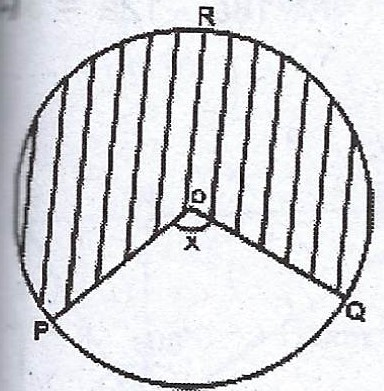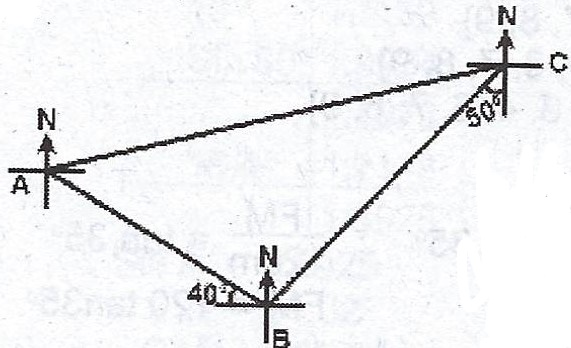### Mathematics Past Questions

3716

(a) The scale of a map is 1 : 20,000. Calculate the area, in square centimetres, on the map of a forest reserve which covers 85$$km^{2}$$.

(b) A rectangular playing field is 18m wide. It is surrounded by a path 6m wide such that its area is equal to the perimeter of the path. Calculate the length of the field.

(c)The diagram shows a circle centre O. If < POQ = x°, the diameter of the circle is 7 cm and the area of the shaded portion is 27.5$$cm^{2}$$. Find, correct to the nearest degree, the value of x. [Take $$\pi = \frac{22}{7}$$].

3717

(a) Madam Kwakyewaa imported a quantity of frozen fish costing GH¢ 400.00. The goods attracted an import duty of 15% of its cost. She also paid a sales tax of 10% of the total cost of the goods including the import duty and then sold the goods for GH¢ 660.00. Calculate the percentage profit.

(b) In a school, there are 1000 boys and a number of girls. The 48% of the total number of students that were successful in an examination was made up of 50%of the boys and 40% of the girls. Find the number of girls in the school.

3718

Using ruler and a pair of compasses only,

(a) construct (i) a quadrilateral PQRS with /PS/ = 6 cm, < RSP = 9 cm, /QR/ = 8.4 cm and /PQ/ = 5.4 cm;   (ii) the bisectors of < RSP and < SPQ to meet at X ;                         (iii) the perpendicular XT to meet PS at T.

(b) Measure /XT/.

3719In the diagram, /AB/ = 8 km, /BC/ = 13 km, the bearing of A from B is 310° and the bearing of B from C is 230°. Calculate, correct to 3 significant figures,

(a) the distance AC ;

(b) the bearing of C from A ;

(c) how far east of B, C is.

3720

(a) Copy and complete the table of values for the relation $$y = -x^{2} + x + 2; -3 \leq x \leq 3$$.

 x -3 -2 -1 0 1 2 3 y -4 2 -4

(b) Using scales of 2 cm to 1 unit on the x- axis and 2 cm to 2 units on the y- axis, draw a graph of the relation $$y = -x^{2} + x + 2$$.

(c) From the graph, find the : (i) minimum value of y ; (ii) roots of equation $$x^{2} - x - 2 = 0$$ ; (iii) gradient of the curve at x = -0.5.